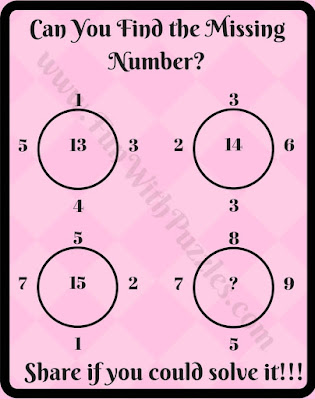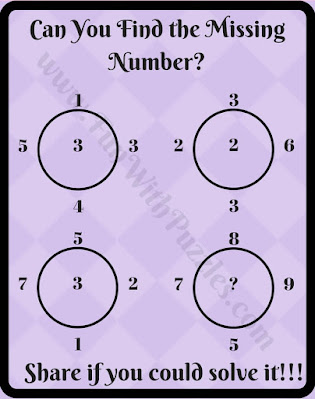Solving mathematical problems is very fun. It becomes more fun when logic is mixed in with mathematical problems. In such problems first one has to find the logical pattern among the given numbers and then has to solve the given problem mathematically. Here we are posting such Math Logic Puzzles Questions which will be very useful for school-going students. Hope school-going kids and teens will have fun solving these puzzles.

## How to Solve Maths Logic Circle Puzzle Questions

In these Picture Maths Puzzles, four circles are given and each circle is having four numbers around it and there is one number in the middle of the circle. This middle circle number has some logical and mathematical relationship with the numbers around the circle. One has to find these logical relations among the given numbers and then has to find which number will replace the question mark.
These puzzles have been arranged from easy to difficult levels. The first few puzzles are straightforward, and the last few are tough. Do post your answers for these puzzles in the comment section of this post and also provide feedback for these puzzles, so that we can improve our future posts :)1. Easy math puzzle to find the missing number in the circle2. Can you solve this easy circle math riddle?3. Find the missing number circle brain teaser4. Tough math circle puzzle question5. Tricky math circle puzzle question

## Answers to Maths Logic Puzzle Questions

Don't forget to check the following brain teasers, riddles, and puzzles.

## Easy Mathematical Brain Teasers with Answers

It contains many interesting Mathematical Brain Teasers to twist your brain.

Here, you can find many different Fun Brain Teasers which challenge your mind.

## Mathematics Logic Brain Cracking Problems with Answers

In these Puzzles, Mathematics and Logic are mixed. You have to solve the mathematical equations in these puzzles which are not correct Mathematically but these equations are correct logically.

## Math Picture Brain Teasers with Answers and Explanation

This contains a mix of different Mathematical Picture Puzzles.

Unknown said...

WHAT IS THE ANSWER FOR THE PINK ONE

Rajesh Kumar said...

Answer link is given after the 5th puzzle image. You can check the answers for all these maths logic circle puzzle questions.

Unknown said...

My name is Shruti